# 第 4 章 Sampling And Sample Distribution

## 4.1 抽样方法

• 简单随机抽样——从总体N个单位里抽出n个单位作为样本（可以重复抽样，也可以不重复抽样），最常用的抽样方式，参数估计和假设检验主要依据的就是简单随机样本。
• 系统抽样——将总体中的所有单位(抽样单位)按一定顺序排列， 在规定的范围内随机地抽取一个单位作为初始单位， 然后按事先规定好的规则确定其他样本单位（先从数字1到k之间随机抽取一个数字r作为初始单位，以后依次取r+k， r+2k…等单位）。
• 分层抽样——将总体单位按某种特征或某种规则划分为不同的层(Strata)， 然后从不同的层中独立、 随机地抽取样本。
• 整群抽样——将总体中若干个单位合并为组(群)， 抽样时直接抽取群， 然后对中选群中的所有单位全部实施调查。
• 多阶段抽样——先抽取群， 但并不是调查群内的所有单位， 而是再进行一步抽样，从选中的群中抽取出若干个单位进行调查（群是初级抽样单位，第二阶段抽取的是最终抽样单位。将该方法推广， 使抽样的段数增多， 就称为多阶段抽样）

• 方便抽样——研究员依据方便的原则选取对应的样本。
• 判断抽样——研究员根据自己的判断选择样本。
• 自愿样本——被调查者自愿参加调查提供信息。举个跟地学相关的例子——志愿地理信息（Volunteer Geographcial Information,VGI），是指利用工具创建、组装和传播个人资源提供的地理数据，像社交媒体中的签到。
• 滚雪球抽样——首先选择一组进行调查，让调查者提供另外一些属于调查总体的调查对象，然后持续下去
• 配额抽样——先将体中的所有单位按一定的标志（变量） 分为若干类， 然后在每个类中采用方便抽样或判断抽样的方式选取样本单位。

#N表示总体的数据，n为抽样单位，replace=FALSE代表不重复抽样，replace=TRUE代表重复抽样
n <- sample(N,n,replace = FALSE)

## 4.2 正态分布

• 描述连续型随机变量的最重要的分布
• 许多现象都可以由正态分布来描述
• 可用于近似离散型随机变量的分布
• 经典统计推断的基础

$f(x)=\frac{1}{\sigma\sqrt{2\pi}}e^{-\frac{1}{2}(\frac{x-\mu}{\sigma})^2} -\infty<x<\infty$

• 关于x=$$\mu$$的钟形对称性质，峰值在x=$$\mu$$处。
• 均值和标准差一旦决定，该分布形式也就决定了。
• 均值决定分布函数位置，标准差决定函数的扁平程度。
• X轴两侧无限延伸，f(x)无限逼近x轴,但理论上不可能相交。
• 正态随机变量在特定区间上的取值概率由正态曲线下的面积给出，而且其曲线下的总面积等于1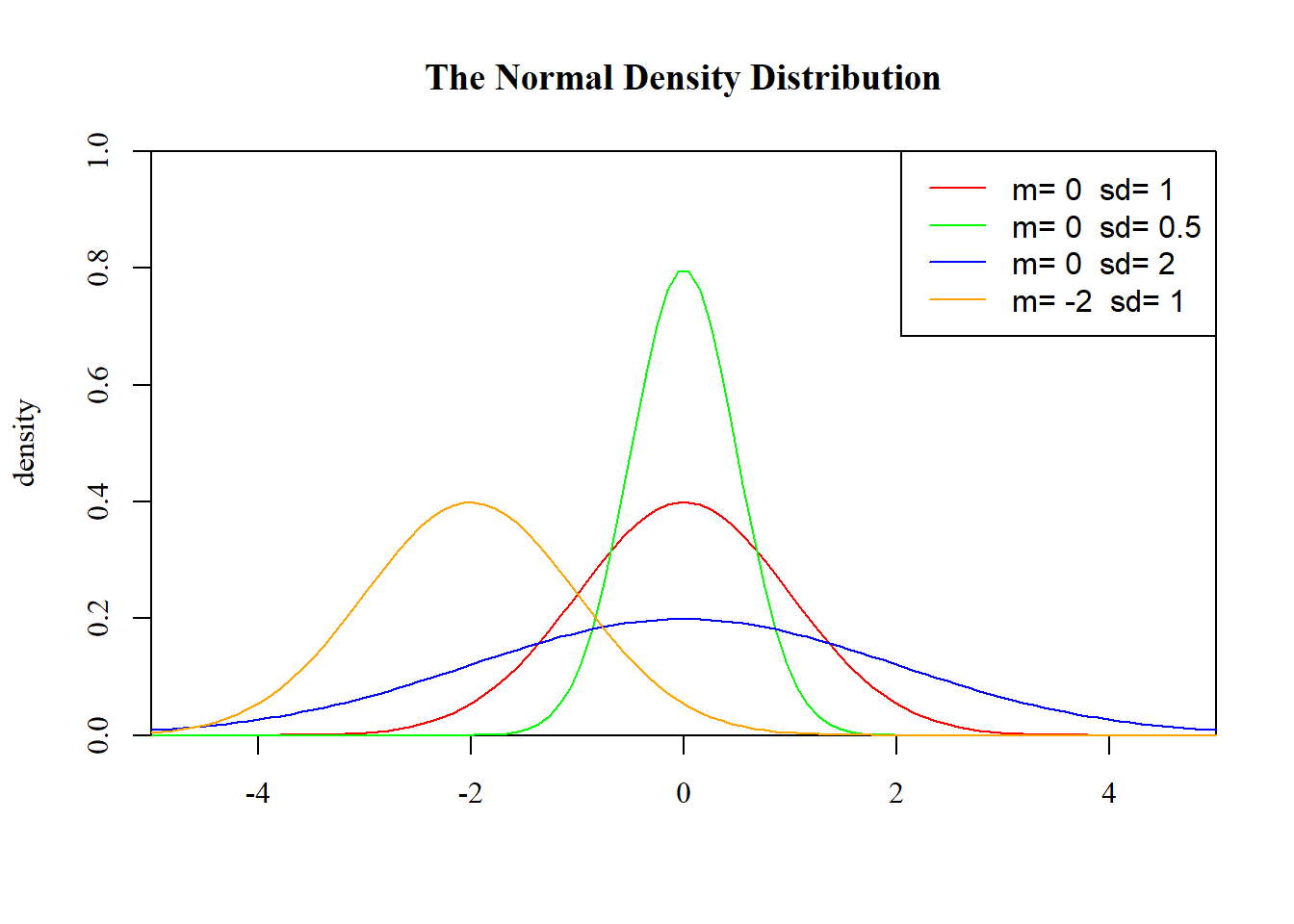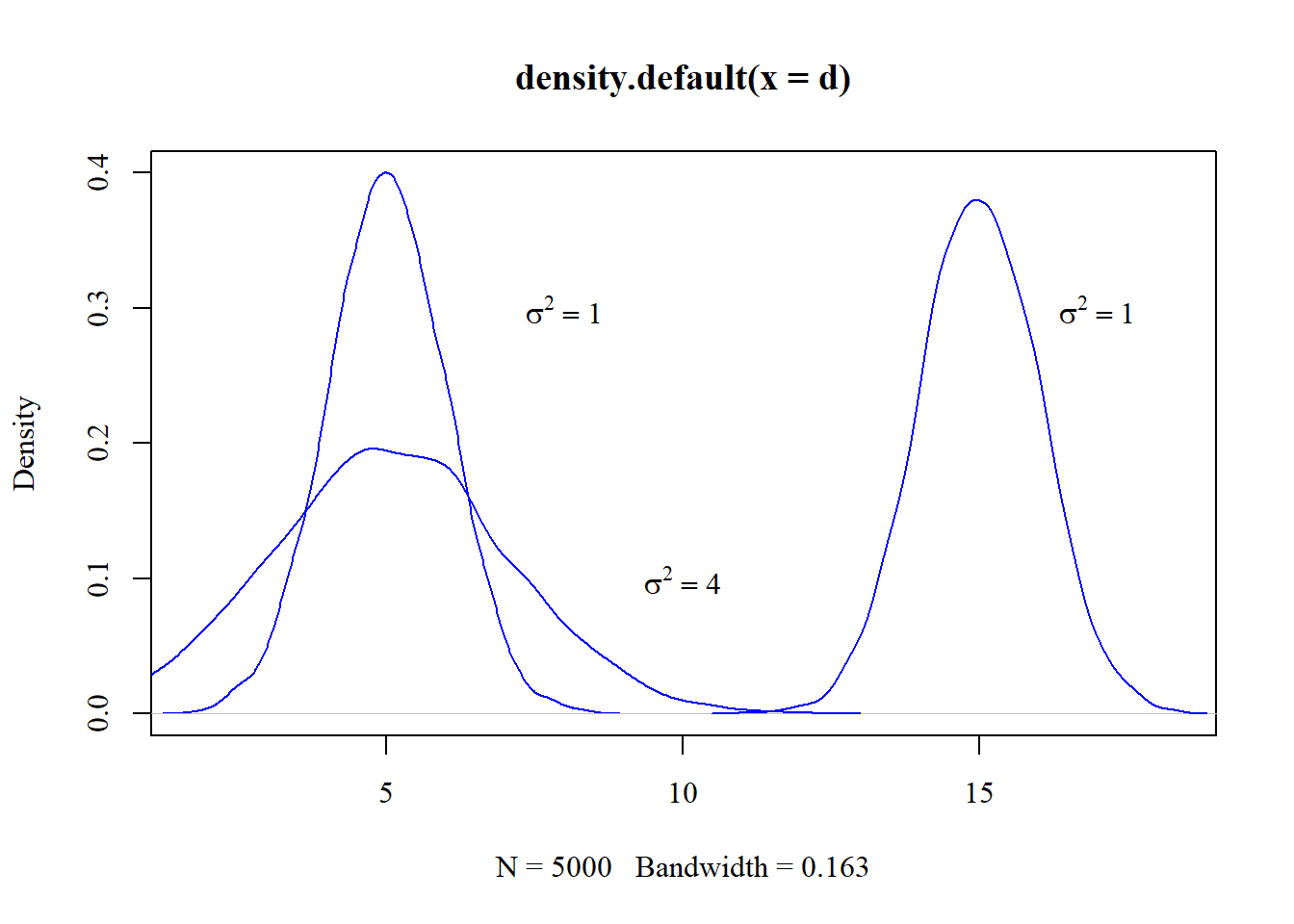• 对数据画出频数分布的直方图或茎叶图（若数据近似服从正态分布， 则图形的形状与上面给出的正态曲线应该相似）。
• 求出样本数据的四分位差和标准差， 然后二者计算比值。 若数据近似服从正态分布，则有 $Q_d/s\approx1/3$
• 拟合优度检验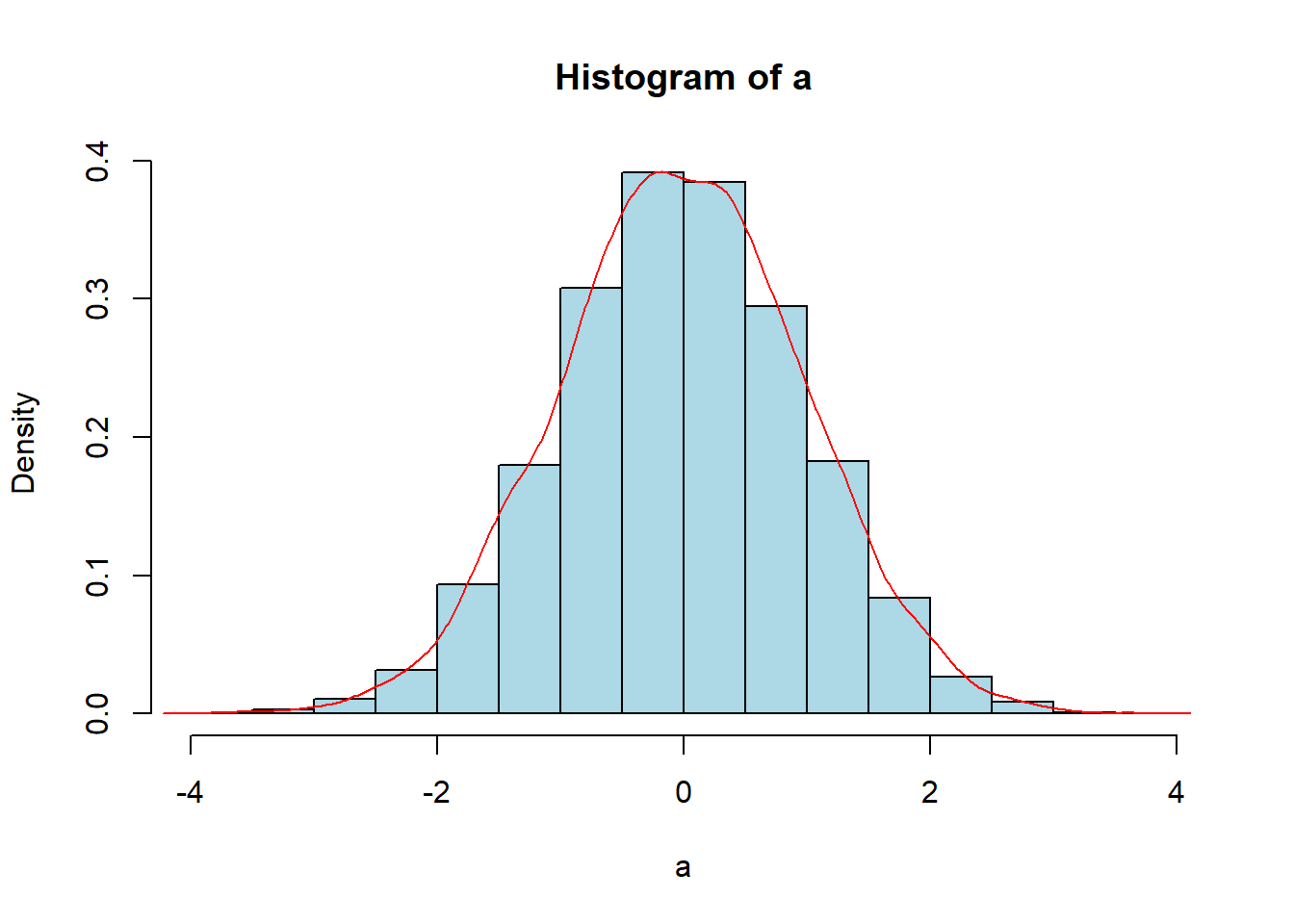Normaltestindex <- function(x) {
q = fivenum(x)
Qd = q - q
s = sd(x)
Normaltestindex = Qd/s
cat("The Qd/s", Normaltestindex)
}

## 4.3 三种不同性质的分布

• 随机性：总体中每个个体都有同等机会被选到样本中,即$$X_i$$与X同分布
• 独立性：样本中每个个体的选取不影响其他个体的选取，即$$X_1,X_2, \cdots ,X_n$$是相互独立的随机变量

• 总体分布——总体中各元素的观察值所形成的分布，分布通常是未知的，可以假定它服从某种分布。
• 样本分布——一个样本中各观察值的分布，也称经验分布，当样本容量 n 逐渐增大时，样本分布逐 渐接近总体的分布。
• 抽样分布——样本统计量的概率分布， 是一种理论分布，又称为诱导分布，在重复选取容量为n 的样本时，由该统计量的所有可能取值形成的相对频数分布，随机变量是样本统计量（样本函数，如样本均值，样本比例，样本方差等），结果来自容量相同的所有可能样本，提供了样本统计量长远而稳定的信息，是进行推断的理论基础，也是抽样推断科学性的重要依据（ 点估计、 置信区间、假设推断等）。

Populationsd <- function(x){
n = length(x)
m = mean(x)
Psd = sqrt(sum((x-m)^2)/n)
cat("The Standard deviation of Population : ", Psd)
}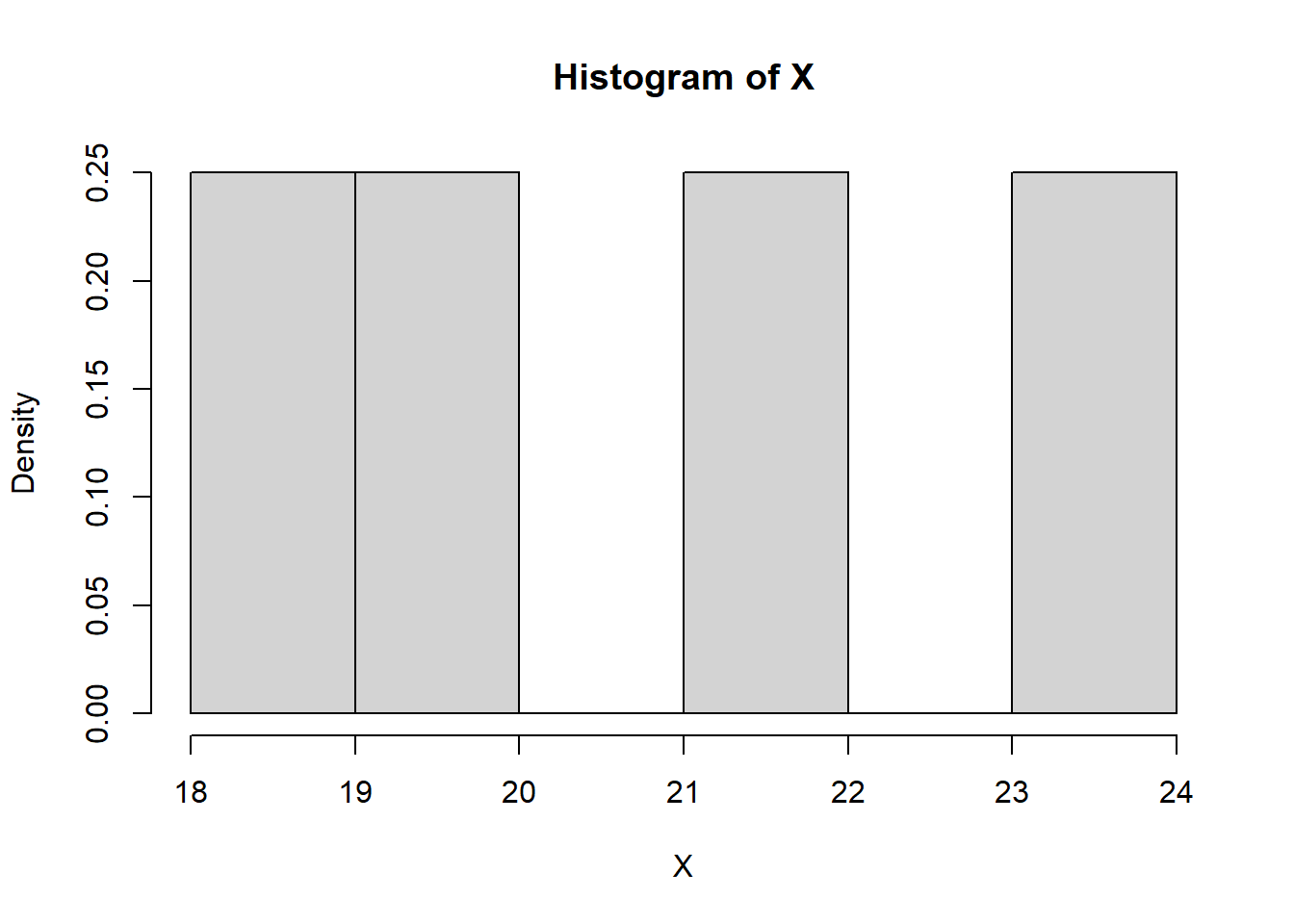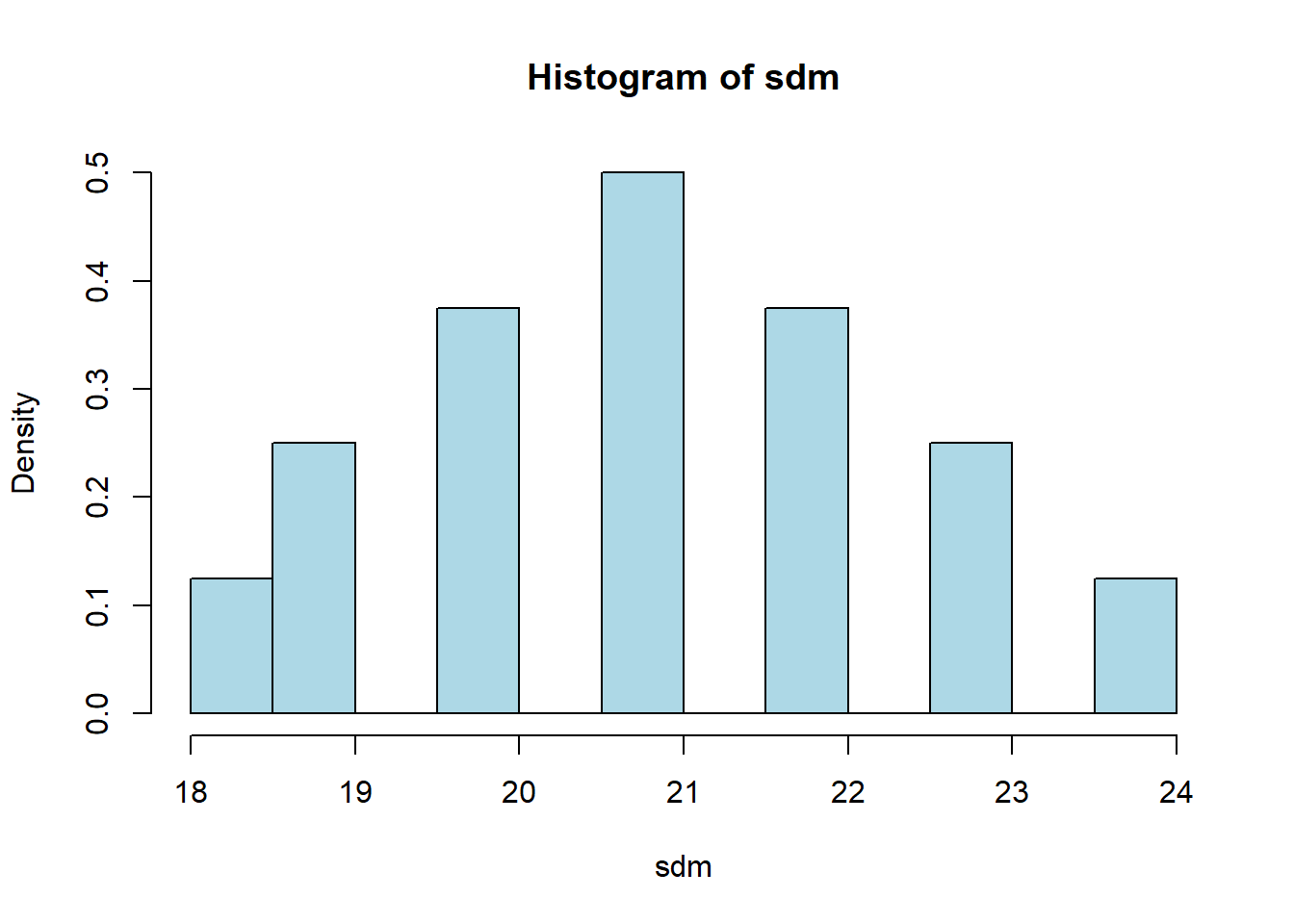• 精确抽样分布：当总体分布已知时，如果对任一自然数都能导出统计量分布的显示表达式，这样的抽样分布称为精确抽样分布（对小样本的统计推断特别有用，大多数是在正态总体下得到的， t分布、 F分布等）。
• 渐近抽样分布：样本量无限大时统计量的极限分布（大样本问题）。
• 近似抽样分布：注意获得近似分布的条件（用统计量的前二阶矩当作正态分布的前二阶矩获得 正态近似，随机模拟法获得统计量的近似分布）。

## 4.4 一个总体样本统计量的抽样分布

• 正态总体均值抽样分布——精确分布（均值无偏）。
• 样本均值的中心极限定理——渐进分布。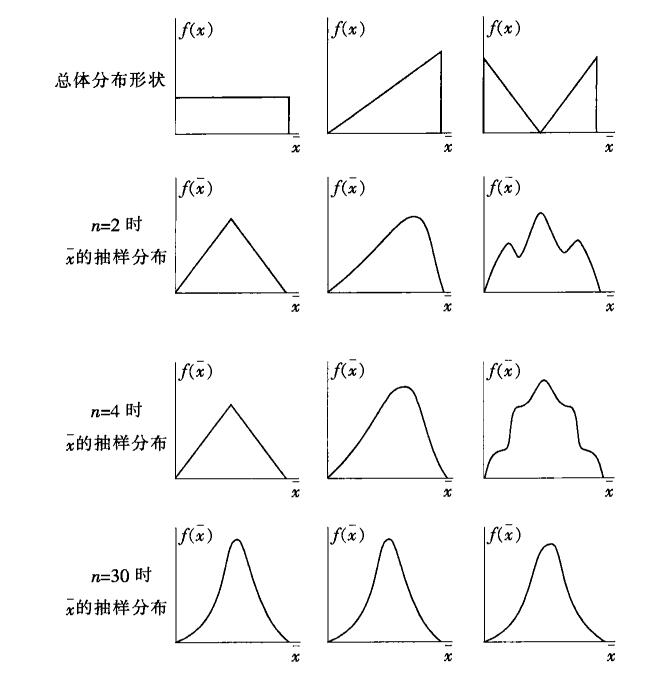$E(\bar x) =\mu$

$\sigma_{\bar x}^2=\frac{\sigma^2}{n}$ （重复抽样）,

$\sigma_{\bar x}^2=\frac{\sigma^2}{n}\frac{N-n}{N-1}$（样本总体有限，且$$n\ge 5\%N$$不重复抽样）

• 一种理论概率分布。
• 当样本容量很大时（满足np≥5, n(1-p)≥5)，样本比例的抽样分布可用正态分布近似。
• 推断总体比例p的理论基础。

$E(\bar p) =p$

$\sigma_{\bar x}^2=\frac{p(1-p)}{n}$ （重复抽样）, $\sigma_{\bar x}^2=\frac{p(1-p)}{n}\frac{N-n}{N-1}$（不重复抽样）

• 分布的变量值始终为正
• 分布的形状取决于其自由度n的大小， 通常为不对称的单峰右偏（ 正偏） 分布， 但随着自由度的增大逐渐趋于对称， 当n>30时， 接近正态分布。
• 期望为： $$E(\chi^2)=n$$，方差为： $$D(\chi^2)=2n$$(n为自由度)
• 可加性：若U和V为两个独立的$$\chi^2$$分布随机变量，$$U\sim \chi^2(n_1)$$$$V\sim \chi^2(n_2)$$, 则U+V这一随机变量服从自由度为$$n_1+n_2$$$$\chi^2$$分布• 自由度为1的t 分布为柯西分布，期望值不存在。
• n>1时，期望值为0。
• n>2时，方差存在，为n/(n-2)。
• 随着自由度的增大，分布也逐渐趋于标准正态分布。（t 分布的极限为标准正态分布，当n>30时， t 分布可用标准正态分布近似）

t分布的性质可以根据这张图来看。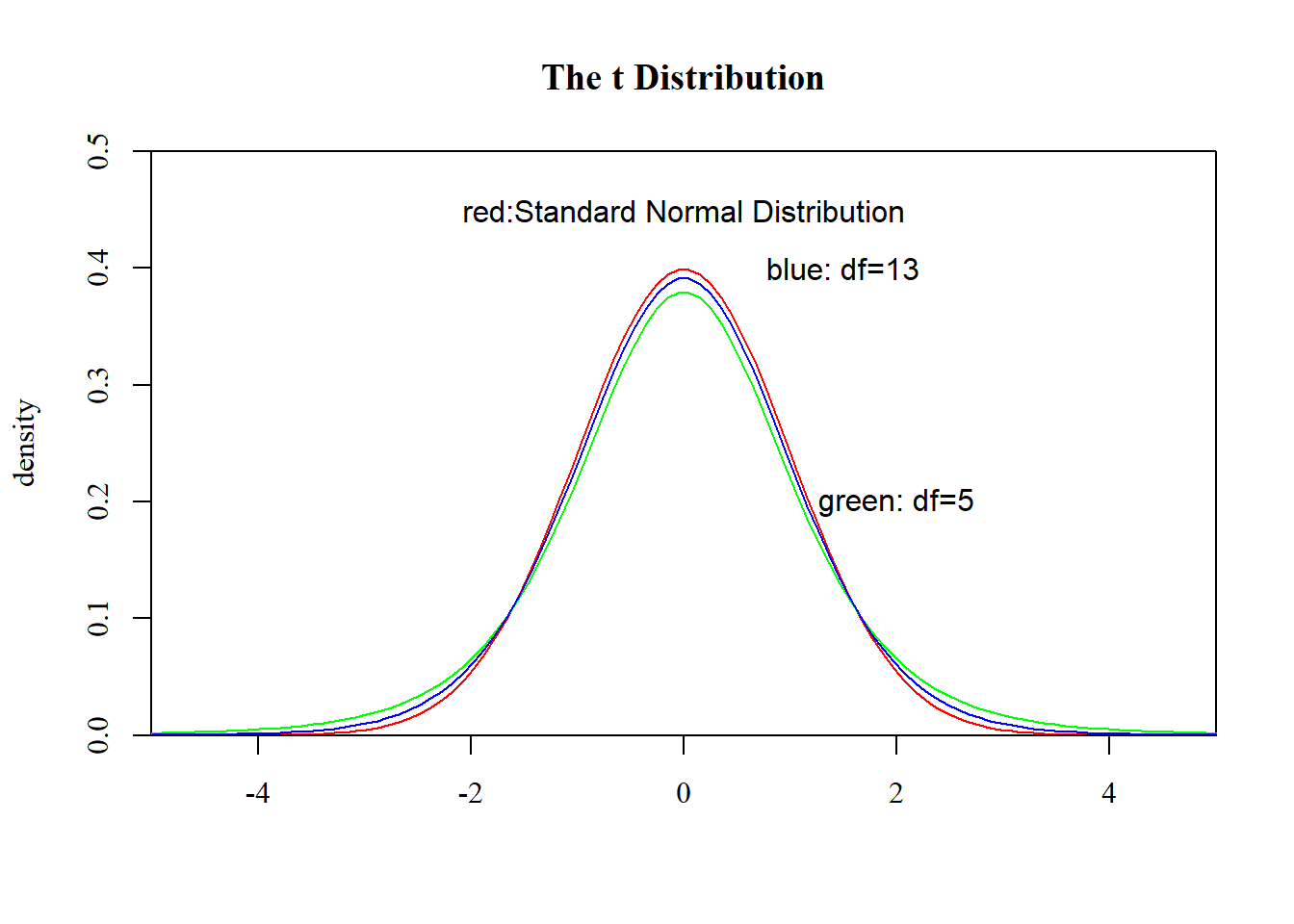t分布的应用是在求样本均值与样本标准差之比上，样本均值与样本标准差之比的分布为：$$t=\frac{\bar x-\mu}{s/\sqrt{n}}\sim t(n-1)$$，自由度为(n-1)的t分布。

## 4.5 两个总体样本统计量的抽样分布

• 两个总体均为正态分布，即$$X_1\sim N(\mu_1,\sigma_1^2),X_2\sim N(\mu_2,\sigma_2^2)$$
• 两个样本均值之差的抽样分布服从正态分布，其分布的数学期望为两个总体均值之差：$$E(\bar x_1-\bar x_2)=\mu_1-\mu_2$$
• 方差为各自的方差之和：$$\sigma_{\bar x_1-\bar x_2}^2=\frac{\sigma_1^2}{n_1}+\frac{\sigma_2^2}{n_2}$$

• 两个总体都服从二项分布。
• 分别从两个总体中抽取容量为$$n_1$$$$n_2$$的独立样本，当两个样本都为大样本时，两个样本比例之差的抽样分布可用正态分布来近似。
• 分布的数学期望为：$$E(\bar p_1-\bar p_2)=p_1-p_2$$
• 方差为各自方差之和：$$\sigma_{\bar p_1-\bar p_2}^2=\frac{p_1(1-p_1)}{n_1}+\frac{p_2(1-p_2)}{n_2}$$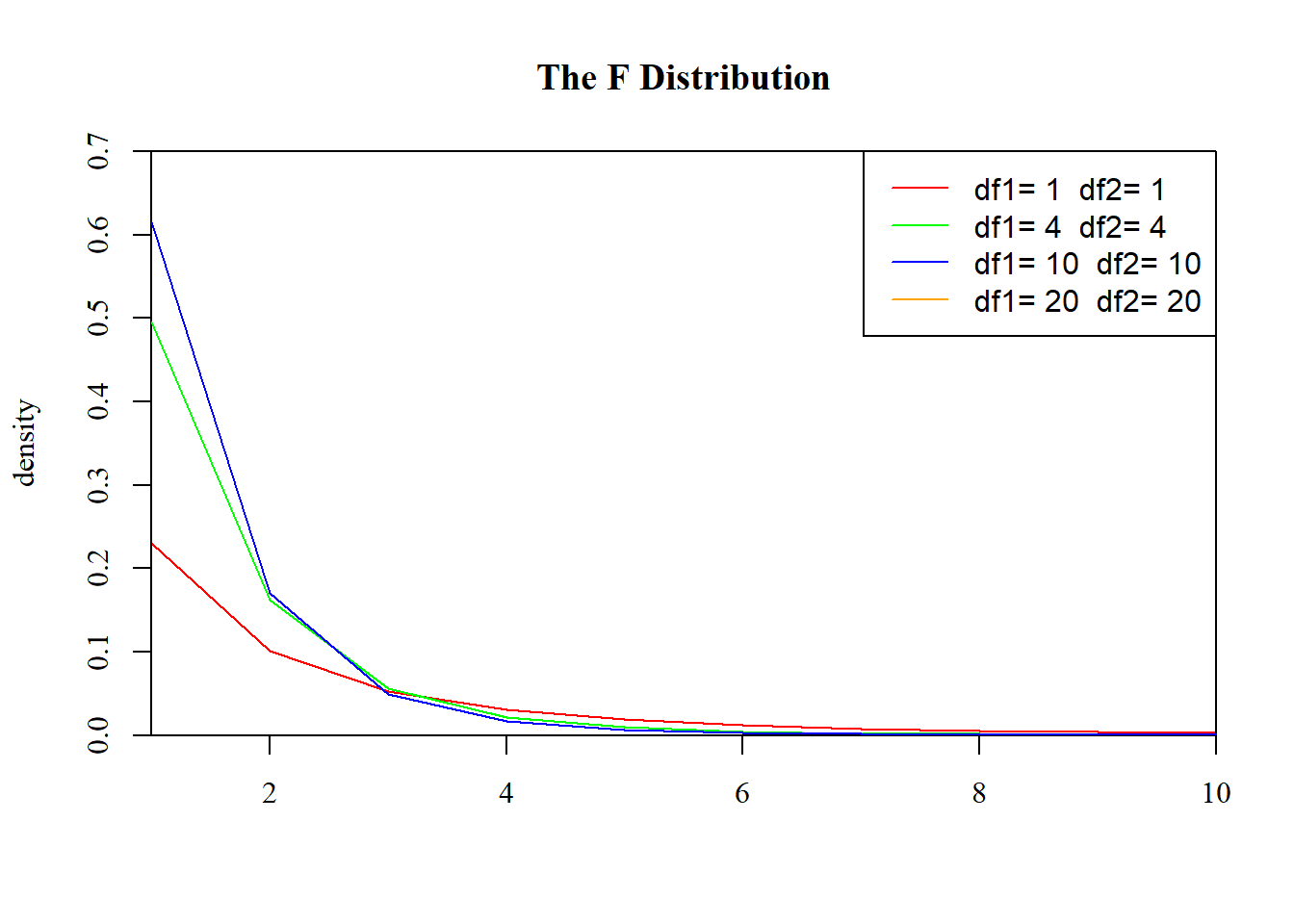## 4.6 附录

$\bar X=\frac{1}{n} \sum_{i=1}^n{X_i}$

$S^2=\frac{1}{n-1} \sum_{i=1}^n(X_i-\bar X)^2$

$S=\sqrt{\frac{1}{n-1} \sum_{i=1}^n(X_i-\bar X)^2}$

$A_k=\frac{1}{n} \sum_{i=1}^nX_i^k(k=1,2,\cdots)$

$B_k=\frac{1}{n} \sum_{i=1}^n(X_i-\bar X)^k(k=1,2,\cdots)$

$\bar x=\frac{1}{n} \sum_{i=1}^n{x_i}$

$s^2=\frac{1}{n-1} \sum_{i=1}^n(x_i-\bar x)^2$

$a_k=\frac{1}{n} \sum_{i=1}^nx_i^k(k=1,2,\cdots)$

$b_k=\frac{1}{n} \sum_{i=1}^n(x_i-\bar x)^k(k=1,2,\cdots)$